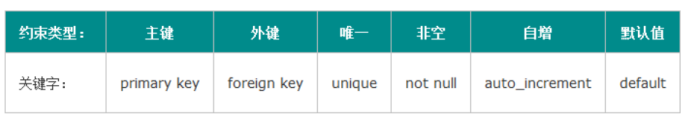﻿ Day29

### Day29

2021-11-4 22:29:51

1. SQL

SQL，它的全称叫Structured Query Language，结构化的查询语言。之所以出现这个东西，是为了统一/屏蔽不同数据库厂商生产的数据库产品之间的差异。

SQL定义了一系列标准和规范,数据库厂商也需要按照这个规范来,当然会有一些细小的差别,相比没有规范来说,要好很多

2.DDL

2.1 概述

DDL : Data Definition Language 数据库定义语言

2.2 DDL基础

-- alter table 表名 rename 新表名

-- alter table 表名 change 列名 新列名 数据类型;

-- alter table 表名 add 列名 类型;  把该列添加到尾部

-- alter table 表名 add 列名 类型 after 已有列名;  把该列添加到指定列的后面

-- alter table 表名 add 列名 类型 first;  把该列添加到首部

-- alter table 表名 drop 列名;

2.3 DDL增强

2.3.1 约束分类create table person(

id int ,

name varchar(100),

income decimal(18,2),

primary key (id,name)

);

create table person1(

id int ,

name varchar(100),

income decimal(18,2),

primary key (id)

);

create table person2(

id int primary key,

name varchar(100) ,

income decimal(18,2)

);

create table person3(

id int ,

name varchar(100),

income decimal(18,2)

);

alter table person3 add primary key(id);

create table person4(

id int auto_increment ,

name varchar(200),

primary key(id)

);

create table person5(

id int ,

name varchar(200),

primary key(id)

);

create table person6(

id int auto_increment ,

name varchar(200),

primary key(id)

);

create table teacher(

id int ,

name varchar(20),

primary key (id)

);

create table student (

id int ,

name varchar(20),

teacher_id int ,

primary key (id),

foreign key (teacher_id) references teacher(id)

);

create table student1 (

id int ,

name varchar(20),

teacher_id int,

primary key (id)

);

create table teacher1(

id int ,

name varchar(20),

primary key (id)

);

create table temp (

id int ,

`name` varchar(20),

unique(id)

);

create table temp (

id int  unique ,

`name` varchar(20)

);

create table temp1 (

id int ,

`name` varchar(20)

);

alter table temp1 add unique (id);

create table temp2(

id int not null,

`name` varchar(30) default  'abc',

sex varchar(10) not null default '男'

);

create table temp3(

id int,

`name` varchar(30) ,

sex varchar(10)

);

3.条件判断

create table student(

id int,

`name` varchar(30),

score decimal(18,2)

);

### and

select 列限定 from 表限定 where A表达式 and B表达式;

### or

select 列限定 from 表限定 where A表达式 or B表达式;

> : 大于

< : 小于

>= : 大于等于

<= : 小于等于

= : 相等

<> : 不等于

### between  and

select 列限定 from 表限定 where 列名 between 值1 and 2;

### In

select 列限定 from 表限定 where 列名 in(值1,值2....);

### 模糊查询like

_ 匹配单个任意字符

select 列限定 from 表限定 where 列名 like  '值' ;

4.Order by排序

select 列限定 from 表限定 order by 列名 asc/desc;

Asc : 升序

Desc : 降序

### Limit

select 列限定 from 表限定 limit 条数;

select 列限定 from 表限定 limit 开始值(不包含) ,条数;

5.单表查询(组函数)

MYSQL中有一类特殊的函数，用于统计，或者分组统计,

### 常用组函数有 :

count(*) : 总条数

max(字段名) : 最大值

min(字段名) : 最小值

avg(字段名) : 平均值

sum(字段名) : 总和

select  count(*),max(字段名),min(字段名)... from 表名 group by 字段名;

### Having 过滤

select teacher_id, avg(score) as avg from student group by teacher_id having avg > 60;

6.Union与 union all

Union 会去除重复项

Union all 不会去除重复项

select * from student where teacher_id=1;

select * from student where score > 60;

// 用 or 实现

select * from student where teacher_id=1 or score > 60;

// 用 union实现

select * from student where teacher_id=1

union

select * from student where score > 60;

select * from student where teacher_id=1

union all

select * from student where score > 60;